# KSEEB Solutions for Class 9 Maths Chapter 11 Areas of Parallelograms and Triangles Ex 11.3

## Karnataka Board Class 9 Maths Chapter 11 Areas of Parallelograms and Triangles Ex 11.3

Question 1.
In Fig., E is any point on median AD of a ∆ABC. Show that ar. (ABE) = ar.(ACE).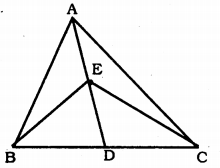Solution:
Data: E is any point on Median AD of an ∆ABC.
To Prove: ar.(∆ABE) = ar. (∆ACE)
Proof: In ∆ABC, AD is the median.
∴ ∆ABD = ∆ACD ……….. (i)
In ∆EBC, DE is the median.
∴ ∆EBC = ∆ECD …………. (ii)
Subtracting (ii) from (i),
∆ABD – ∆EBD = ∆ACD – ∆ECD
∴ ar. (∆ABE) = ar. (∆ACE).

Question 2.
In a triangle ABC, E is the mid-point of median AD. Show that ar.(∆BED) = $$\frac{1}{4}$$ ar.(∆ABC).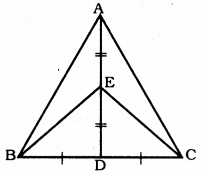Solution:
Data: In a triangle ABC, E is the midpoint of median AD.
To Prove: ar.(∆BED) = $$\frac{1}{4}$$ ar.(∆ABC)
Proof: D is the midpoint of BD.
∴ BD = $$\frac{1}{2}$$ BC ……… (i)
E is the midpoint of AD.
∴ DE = $$\frac{1}{2}$$ AD …………(ii)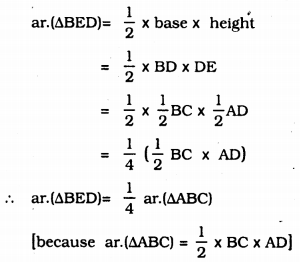Question 3.
Show that the diagonals of a parallelogram divide it into four triangles of equal area.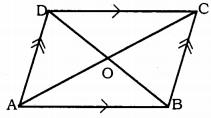Solution:
Data: Diagonals of ABCD, AC and BD intersect at ‘O’.
To Prove: ar.(∆AOD) = ar.(∆DOC) = ar.(∆COB) = ar.(∆AOB)
Proof: ‘O’ is the mid-point of diagonals AC and BD.
DO is the median of ∆ADC.
∴ ar.(∆AQD) = ar.(∆DOC) ……….. (i)
CO is the median of ∆DCB.
∴ ar. (∆DOC) = ar.(∆BOC) ……….. (ii)
BO is the median of ∆ABC.
∴ ar.(∆BOC) = ar.(∆AOB) …………. (iii)
AO is the median of ∆ADB.
∴ ar.(∆AOB) = ar.(∆AOD) …………. (iv)
From (i), (ii), (iii) and (iv),
ar. (∆AOD) = ar. (∆DOC) = ar. (∆COB) = ar. (∆AOB)
∴ The diagonals of a parallelogram divide it into four triangles of equal area.

Question 4.
In Fig., ABC and ABD are two triangles on the same base AB. If line- segment CD is bisected by AB at O, show that ar.(∆ABC) = ar.(∆ABD).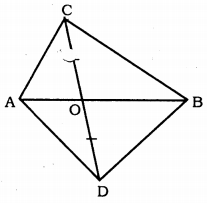Solution:
Data ∆ABC and ∆ABD are two triangles on the same base AB.
Line segment CD bisected by AB at O’.
To Prove: ar.(∆ABC) = ar.(∆ABD)
Prove: AB bisects line segment CD at O’.
∴ OC = OD
In ∆ADC, AO is the median on CD.
∴ ar.(∆AOD) = ar.(∆AOC) …………… (i)
In ∆BDC, BO is the median on CD.
∴ ar.(∆BOD) = ar.(∆BOC) ……………. (ii)
ar.(∆AOD) + ar.(∆BOD) = ar.(∆AOC)+ ar.(∆BOC)
∴ ar.(∆ABD) = ar.(∆ABC)

Question 5.
D, E, and F are respectively the midpoints of the sides BC, CA, and AB of an ∆ABC. Show that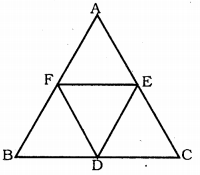(i) BDEF is a parallelogram.
(ii) ar.(∆DEF) = $$\frac{1}{4}$$ ar. (∆ABC)
(iii) ar.(BDEF) = $$\frac{1}{2}$$ ar.(∆ABC).
Solution:
Data : D, E, and F are respectively the mid-points of the sides BC, CA, and AB of an ∆ABC.
To Prove:
(i) BDEF is a parallelogram.
(ii) ar.(ADEF) = $$\frac{1}{4}$$ ar.(∆ABC)
(iii) ar.(BDEF) = $$\frac{1}{2}$$ ar.(∆ABC).
Proof: (i) In ∆ABC, D, E and F are respectively mid-points of the sides BC, CA and AB.
∴ BD = $$\frac{1}{2}$$ BC
and EF = $$\frac{1}{2}$$ BC (mi-point theorem) z .
EF || BC
∴ BD = EF
BD || EF
∴ BDEF is a parallelogram.

(ii) Diagonals of BDEF divides into two congruent triangles.
∴ ∆BDF ≅ ∆DEF ………… (i)
Similarly, in DCEF,
∆DCE ≅ ∆DEF …………. (ii)
Similarly, in AEDF,
∴ ∆AEF ≅ ∆DEF ……….. (iii)
From (i), (ii) and (iii),
ar.(∆BDF) = ar.(∆DEF) = ar.(∆DCE) = ar.(∆AEF)
∴ 4 × ar.(∆DEF) = ar.(∆ABC)
∴ ar.(∆DEF) = $$\frac{1}{4}$$ar.(∆ABC)

(iii) ar.(∆BED) + ar.(∆DCE) + ar.(∆AEF) + ar.(∆DEF) = ar.(∆ABC)
∆BDF + ∆BDF + ∆DEF + ∆DEF = ar.(∆ABC)
2 × ∆BDE + 2 × ∆DEF = ar.(∆ABC)
2(∆BDF + ∆DEF) = ar.(∆ABC)
2 × ar. (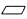BDEF) = ar.(∆ABC)
ar.(BDEF) = $$\frac{1}{2}$$ × ar.(∆ABC)

Question 6.
In Fig., diagonals AC and BD of quadrilateral ABCD intersect at O such that OB = OD. If AB = CD, then show that: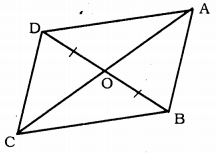(i) ar.(∆DOC) = ar.(∆AOB)
(ii) ar.(∆DCB) = ar.(∆ACB)
(iii) DA || CB or ABCD is a parallelogram.
[Hint: From D and B, draw perpendiculars to AC.]
Solution:
Data: Diagonals AC and BD of quadrilateral ABCD intersect at O such that OB = OD. If AB = CD,
To Prove:
(i) ar.(∆DOC) = ar.(∆AOB)
(ii) ar.(∆DCB) = ar.(∆ACB)
(iii) DA || CB or ABCD is a parallelogram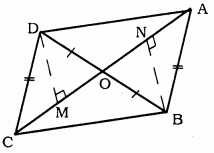Construction: Draw DM ⊥ AC and BN ⊥ AC.
Proof: (i) DM ⊥ CO, BN ⊥ AO.
In ∆DOM and ∆BON,
DO = BO (data)
∠DMO = ∠BNO = 90° (Construction)
∠DOM = ∠BON (Vertically opposite angles)
∴ ∆DOM ≅ ∆BON (AAS Postulate)
∴ DM = BN
∴ ar.(∆DOM) = ar.(∆BON) ………… (i)
In ∆DMC, and ∆BNA,
∠DMC = ∠BNA = 90°
DC = AB (Data)
DM = BN (Proved)
∴ ⊥∆DMC = ⊥∆BNA
∴ ar. (∆DMC) = ar, (∆BNA) ………… (ii)
ar.(∆DOM)+ ar.(∆DMC) = ar.(∆BON) + ar.(∆BNA)
∴ (∆DOC) = (∆AOB) …………….. (iii)

(ii) (∆DOC) = (∆AOB) (Proved)
∆DOC + ∆BOC = ∆AOB + ∆BOC
∴ ∆DCB = ∆ACB.

(iii) From equation (ii), ∆DMC = ∠BNA
∴ ∠DCM = ∠BAN.
These are a pair of alternate angles.
∴ AB || DC
and also AB = DC (Data)
∴ ABCD is a parallelogram OR DA || CB.

Question 7.
D and E are points on sides AB and AC respectively of ∆ABC such that ar.(∆DBC) = ar.(∆EBC). Prove that, DE || BC.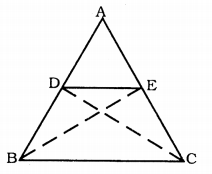Solution:
Data : D and E are mints on sides AB and AC respectively of ∆ABC such that ar.(∆DBC) = ar.(∆EBC).
To Prove DE || BC.
Construction: BE and DC joined.
Proof: ∆DBC and ∆EBC are on base BC.
ar.(∆DBC) = ar. (∆EBC) (Data)
Two triangles on the same base (or equal bases) and between the same parallel are equal in area.
∴ BC || DE.

Question 8.
XY is a line parallel to side BC of a triangle ABC. If BE || AC and CF || AB meet XY at E and F respectively, show that ar.(∆ABE) = ar.(∆ACF).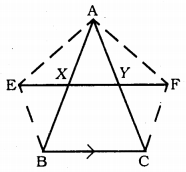Solution:
Data: XY is a line parallel to side BC of a triangle ABC.
If BE || AC and CF || AB meet XY at E and F respectively.
To Prove: ar.(∆ABE) = ar.(∆ACF).
Proof: XY || BC
∴ EY || BC
and BE || AC
BE || YC
∴ BEYC is a parallelogram.
Similarly, BCFX is a parallelogram.
BEYC and BCFX are on base BC and between BC || EF.
∴ ar.(BEYC) = ar.(BCFX) ………….. (i)
Now, ∆ABE and BEYC are on base BE and in between BE || AC.
∴ ar.(∆ABE) = $$\frac{1}{2}$$ ar.(BCYX) …………… (ii)
Similarly, ∆ACF and BCFX are on base CF and in between CF || AB.
∴ ar.(∆ACF) = $$\frac{1}{2}$$ ar.(imggrectBCFX) …………….. (iii)
Comparing (i), (ii) and (iii),
∴ ar. (∆ABE) = ar. (∆ACF)

Question 9.
The side AB of a parallelogram ABCD is produced to any point P. A line through A and parallel to CP meets CB produced at Q and then parallelogram PBQR is completed. Show that ar.(ABCD) = ar(PBQR).
[Hint: Join AC and PQ. Now compare ar.(∆ACQ) and ar.(∆APQ)]
Solution:
Data: The side AB of a parallelogram ABCD is produced to any point P. A line through A and parallel to CP meets CB produced at Q and then parallelogram PBQR is completed.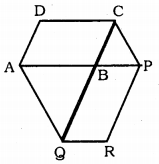To Prove: ar.(ABCD) = ar.(PBQR)
Construction: AC and CQ are joined.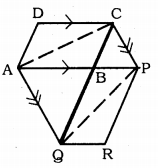Proof: ∆ACQ and ∆APQ are on base AQ and in between AQ || CP.
∴ (∆ACQ) = (∆APQ)
Now, ∆ACQ – ∆ABQ = ∆APQ – ∆ABQ
∴ ∆ABC = ∆QBP ………….. (i)
Diagonals divides quadrilateral into two congruent triangles.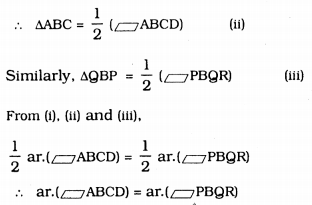Question 10.
Diagonals AC and BD of a trapezium ABCD with AB || DC, intersect each other at ‘O’. Prove that ar.(∆AOD) = ar.(∆BOC).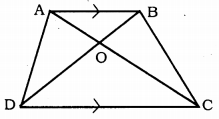Solution:
Data: Diagonals AC and BD of a trapezium ABCD with AB || DC, intersect each other at ‘O’.
To Prove: ar.(∆AOD) = ar.(∆BOC)
Proof: ∆ABD and ∆ABC are on base AB and between AB || DC.
∴ ar. (∆ABD) = ar. (∆ABC)
Subtracting ar.(∆ABO) on both sides,
ar.(∆ABD) – ar.(∆ABO) = ar.(∆ABC) – ar.(∆ABO)
∴ ar.(∆AOD) = ar.(∆BOC).

Question 11.
In fig., ABCDE is a pentagon. A line through B parallel to AC meets DC produced at F. Show that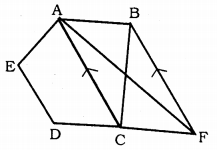(i) ar.(∆ACB) = ar.(∆ACF)
(ii) ar.(∆EDF) = ar.(ABCDE).
Solution:
Data: ABCDE is a pentagon. A line through B parallel to AC meets DC produced at F.
To Prove: (i) ar.(∆ACB) = ar.(∆ACF)
(ii) ar.(AEDF) = ar.(ABCDE)
Proof: (i) ∆ACB and ∆HCF are on base AC and between AC || BF.
∴ ar.(∆ACB) = ar.(∆ACF)
(ii) Now, W(∆ACB) = W(∆ACF) proved,
ar.(∆ACB) + ar.(ACDE) = ar.(∆ACF) + ar.(ACDE)
∴ ar.(ABCDE) = ar.(AEDF)

Question 12.
A villager Itwaari has a plot of land of the shape of a quadrilateral. The Gram Panchayat of the village decided to take over some portion of his plot from one of the corners to construct a Health Centre. Itwaari agrees to the above proposal with the condition that he should be given an equal amount of land in lieu of his land adjoining his plot so as to form a triangular plot. Explain how this proposal will be implemented.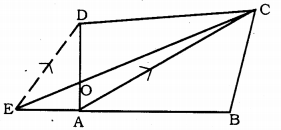Solution:
Let the shape of plot be quadrilateral ABCD. Itwaari agrees to give land as follows:
Diagonal AC of a quadrilateral is joined. BA side of land ABCD is produced to E.
CE and DE || AC are drawn.
Now, ∆ACD and ∆ACE are on same base aC and in between AC || DE.
∴ ar.(∆ACD) = ar.(∆ACE)
Subtracting ar.(∆AOC) on both sides
ar (∆ACD) – ar(∆AOC) = ar(∆ACE) – ar(∆AOC)
ar.(∆DOC) = ar.(∆AOC).
Now, Itwaari agrees to give ADOC to construct a Health Centre. Area of ∆AOE and ∆CEB is remains with Itrwaari.

Question 13.
ABCD is a trapezium with AB || DC. A line parallel to AC intersects AB at X and BC at Y. Prove that area(ADx) = area(ACy). [Hint: Join Cx.)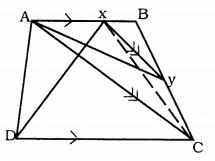Solution:
Data : ABCD is a trapezium with AB || DC. A line parallel to AC intersects AB at x and BC at y.
Construction: Join CX.
Proof: ABCD is a trapezium.
∴ AB || DC
∆ADx and ∆ACx are on same base Ax and in between AB || DC.
∴ (∆ADx) = (∆ACx) ………… (i)
But, AC || xy (Data)
∆ACx and ∆ACy are on same base AC and in between AC || xy.
∴ (∆ACx) = (∆ACy) …………. (ii)
From comparing (i) and (ii),

Question 14.
In Fig., AP || BQ || CR.
Prove that ar.(AQC) = ar.(PBR).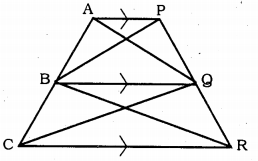Solution:
Data: AP || BQ || CR.
To Prove: ar.(∆AQC) = ar.(∆PBR)
Proof: AP || BQ. CR is a line segment.
∆ABQ and ∆PBQ are on base BQ and in between AP || BQ
ar.(∆ABQ) = ar.(∆PBQ) ………….. (i)
∆BQC and ∆BQR are on base BQ and in between BQ || CR.
∴ ar.(∆BQC) = ar.(∆BQR) ………….. (ii)
ar.(∆ABQ) + ar.(∆BQC) = ar.(∆PBQ) + ar.(∆BQR)
∴ ar.(∆AQC) = ar.(∆PBR).

Question 15.
Diagonals AC and BD of a quadrilateral ABCD intersect at O in such a way that ar.(AOD) = ar.(BOC). Prove that ABCD is a trapezium.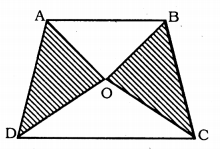Solution:
Data: Diagonals AC and BD of a quadrilateral ABCD intersect at O in such a way that ar.(AOD) = ar.(BOC).
To Prove: ABCD is a trapezium.
Proof: ar.(∆AOD) = ar.(∆BOC) (Data)
ar.(∆AOD) + ar.(∆ODC) = ar.(∆BOC) + ar.(∆ODC)
Area of these triangles are equal, they are on base DC and in between DC, AB straight lines.
∴ DC || AB.
Now, one pair of opposite sides of a quadrilateral are parallel, hence ABCD is a trapezium.

Question 16.
In Fig., ar.(DRC) = ar.(DPC) and ar.(BDP) = ar.(ARC). Show that both the quadrilaterals ABCD and CPR are trapeziums.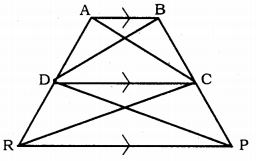Solution:
Data: in fig. ar.(DRC) = ar.(DPC) and
ar.(BDP) = ar.(ARC).
To Prove: (i) ABCD is a trapezium
(ii) DCPR is a trapezium.
Proof: (i) ar.(∆BDP) = ar.(∆ARC) (Data)
ar.(∆DPC) + ar.(∆DCB) = ar.(∆DCR) + ar.(∆DCA)
But, ∆DRC = ∆DPC. (Data)
∴ ar.(∆DCB) = ar.(∆DCA)
These are on same base DC and in between straight lines DC and AB.
∴ DC || AB.
Now, one pair of opposite sides of quadrilateral ABCD are parallel, hence ABCD is a trapezium.

(ii) ∆DRC and ∆DPC are on the same base DC and in between DC and RP and they are equal in area.
∴ DC || RP.
∴ Hence ‘Quadrilateral’ DCPR is a trapezium.